##### Array Operators in PostgreSQL

There are many operators that can be applied to arrays. These operators are used for comparison, containment, overlapping, and concatenation. All of these operations have some unique functionality.

● The comparison operators

● The containment operators

● The overlap operator

● The concatenation operator.

## Array Operators in PostgreSQL

Each array operator serves a unique purpose in the program. Let’s dive into the details of each operator.

### The Comparison Operators

These operators are used to draw a comparison between two arrays. These operators are of two types based on the comparison, which are, equality and ordering.

#### ● Equality Operator

There are two equality operators, “equal to” and “not equal to”. These equality operators perform element-by-element comparisons and return a boolean value, that can either be true or false.

In the case of the “equal to” operator:

● If the query returns “t” which means true after the operator is executed on it, it means that the elements of both the arrays are same.

● If the query returns “f” which means false after the operator is executed on it, it means that the elements of both arrays are not the same.

The case is the opposite for “not equal to”:

● If the query returns “t” which means true after the operator is executed on it, it means that the elements of both arrays are not the same.

● If the query returns “f” which means false after the operator is executed on it, it means that the elements of both arrays are the same.

Now let’s move towards an example so that the concept is more clear:

`SELECT ARRAY['Williams', 'Alex', 'Peter', 'Katherine'] = ARRAY['Katherine', 'Alex'] As is_equal;`

What do you think will the query return? Let's see.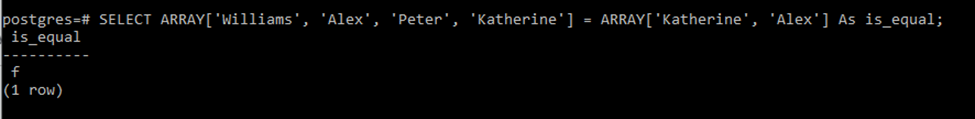The output is “f” which means false. As we have discussed, the equality operators compare array elements, element by element. So if we see elements of both the arrays are not equal i.e. “Williams” is not equal to “Katherine”.

Now if we place the “not equal to” operator between them. It should return “t” because both arrays are not equal.

`SELECT ARRAY['Williams', 'Alex', 'Peter', 'Katherine'] <> ARRAY['Katherine', 'Alex'] As not_equal;`

The output is “t”.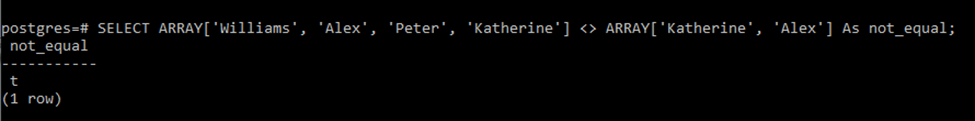Which means both arrays are not equal to each other.

#### ● Ordering Operator

These operators include less than, greater than, less than equal to, and greater than equal to. Following are the queries and their outputs showing how these operators work.

```SELECT ARRAY['Williams', 'Alex', 'Peter', 'Katherine'] > ARRAY['Katherine', 'Alex'] As greater_than,
ARRAY['Williams', 'Alex', 'Peter', 'Katherine'] < ARRAY['Katherine', 'Alex'] As less_than,
ARRAY['Williams', 'Alex', 'Peter', 'Katherine'] >= ARRAY['Katherine', 'Alex'] As   greaterthan_equalto,
ARRAY['Williams', 'Alex', 'Peter', 'Katherine'] <= ARRAY['Katherine', 'Alex'] As   lessthan_equalto;```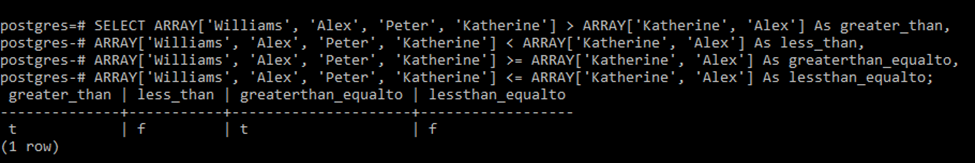### The Containment Operators

The containment operators check whether one array contains elements of another or not. These operators include “@>” and “<@” operators. The “@>” operator checks whether the right array is contained in the left array while the “<@” operator checks whether the left array contains the right one. If they do the queries will return “t”.

Let's have a look at an example of the containment operator so that we have a better understanding.

`SELECT ARRAY['Williams', 'Alex', 'Peter', 'Katherine'] @> ARRAY['Katherine', 'Alex'] As l_contains_r;`

The query above returns the following output: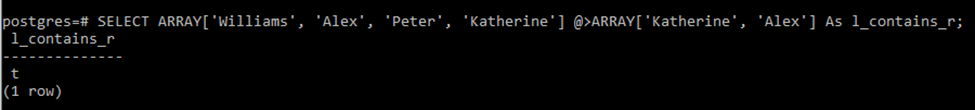And it is clear that the elements of the array on the right side are contained by the array on the left side which is why the query returned true.

Now if we add the “<@” operator between both arrays it should return false because the right array does not contain all the elements of the left array.

`SELECT ARRAY['Williams', 'Alex', 'Peter', 'Katherine'] <@ ARRAY['Katherine', 'Alex'] As r_contains_l;`

Executing the above query gives false: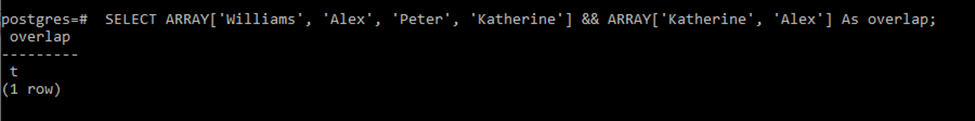This was all about the containment operators.

### The Overlap Operator

The overlap operator is used to find out if both the arrays, operated under this operator, have elements in common or not. In Postgres, the overlap operator is denoted as a “&&” sign.

Let’s execute the same example for the overlap operator.

`SELECT ARRAY['Williams', 'Alex', 'Peter', 'Katherine'] && ARRAY['Katherine', 'Alex'] As overlap;`

So we can clearly see that these arrays have elements in common so the query should return “t”.This was all about the overlap operator. Next, we will move toward the concatenation operator.

### The Concatenation Operator

The concatenation operator is denoted by ”||”. Concatenation links and joins both arrays together. Other than array-to-array concatenation, this operator provides element-to-array and array-to-element concatenation.

We will look at the examples one by one

`SELECT ARRAY['Williams', 'Alex', 'Peter', 'Katherine'] || ARRAY['Katherine', 'Alex'] As concat;`

This is an example of array-to-array concatenation. Let’s see its output: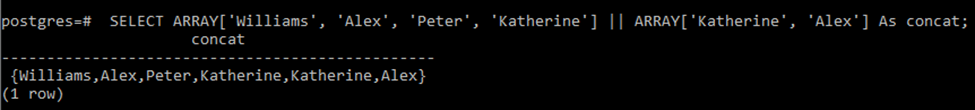The resulting array is a long array that is made by joining the elements of both arrays.

Now consider another case for the array-to-element and element-to-array concatenation.

`SELECT ARRAY[1,2,3] || 4 As concat;`

The above query will result in the following output: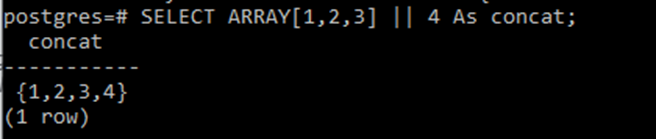Now we will write a query for the element-to-array concatenation:

`SELECT 4 || ARRAY[1,2,3] As concat;`

This will append the element at the start of the array as follows: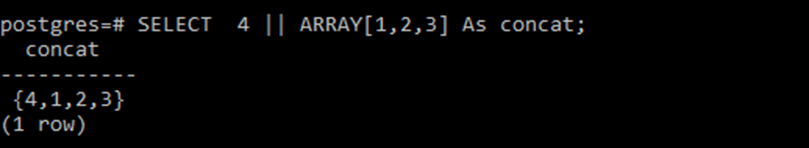So this is how the concatenation operator works.

## Conclusion

We have seen the array operators above in detail. Array operators perform different operations on arrays. These operators are used for comparison, containment, overlapping, and concatenation. The arrays can operate equality, ordering, containment, overlap, and concatenation operators on them.